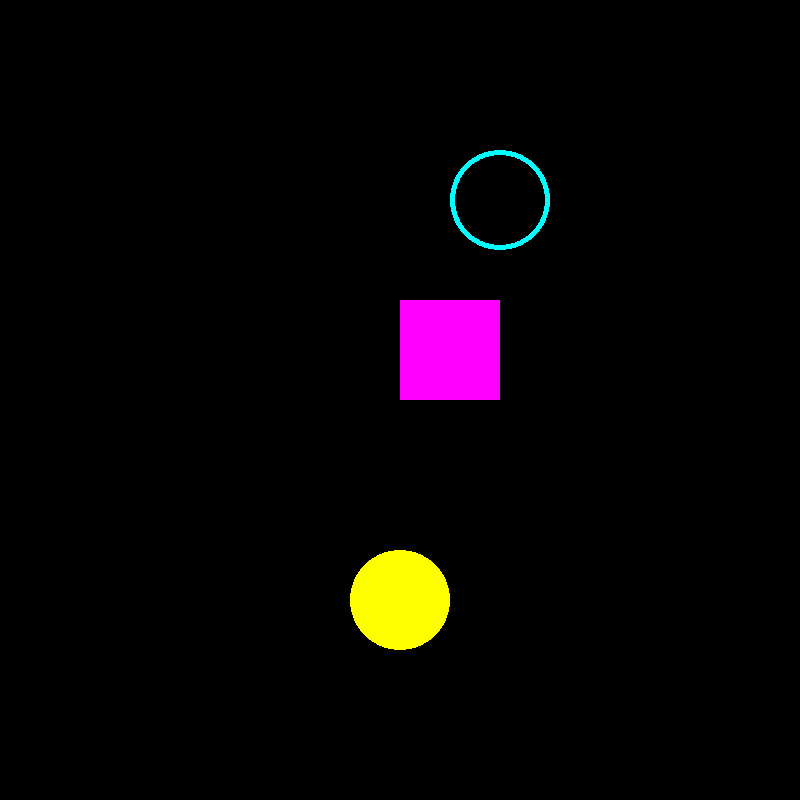# Simple Shapes#

This example shows how to use the UI API. We will demonstrate how to draw some geometric shapes from FURY UI elements.

First, a bunch of imports.

```from fury import ui, window
from fury.data import fetch_viz_icons
```

First we need to fetch some icons that are included in FURY.

```fetch_viz_icons()
```
```Data size is approximately 12KB
Dataset is already in place. If you want to fetch it again please first remove the folder /Users/skoudoro/.fury/icons

({'icomoon.tar.gz': ('https://digital.lib.washington.edu/researchworks/bitstream/handle/1773/38478/icomoon.tar.gz', 'BC1FEEA6F58BA3601D6A0B029EB8DFC5F352E21F2A16BA41099A96AA3F5A4735')}, '/Users/skoudoro/.fury/icons')
```

Let’s draw some simple shapes. First, a rectangle.

```rect = ui.Rectangle2D(size=(100, 100), position=(400, 400), color=(1, 0, 1))
```

Then we can draw a solid circle, or disk.

```disk = ui.Disk2D(outer_radius=50, center=(400, 200), color=(1, 1, 0))
```

```ring = ui.Disk2D(outer_radius=50, inner_radius=45, center=(500, 600), color=(0, 1, 1))
```

Now that all the elements have been initialised, we add them to the show manager.

```current_size = (800, 800)
show_manager = window.ShowManager(size=current_size, title='FURY Shapes Example')